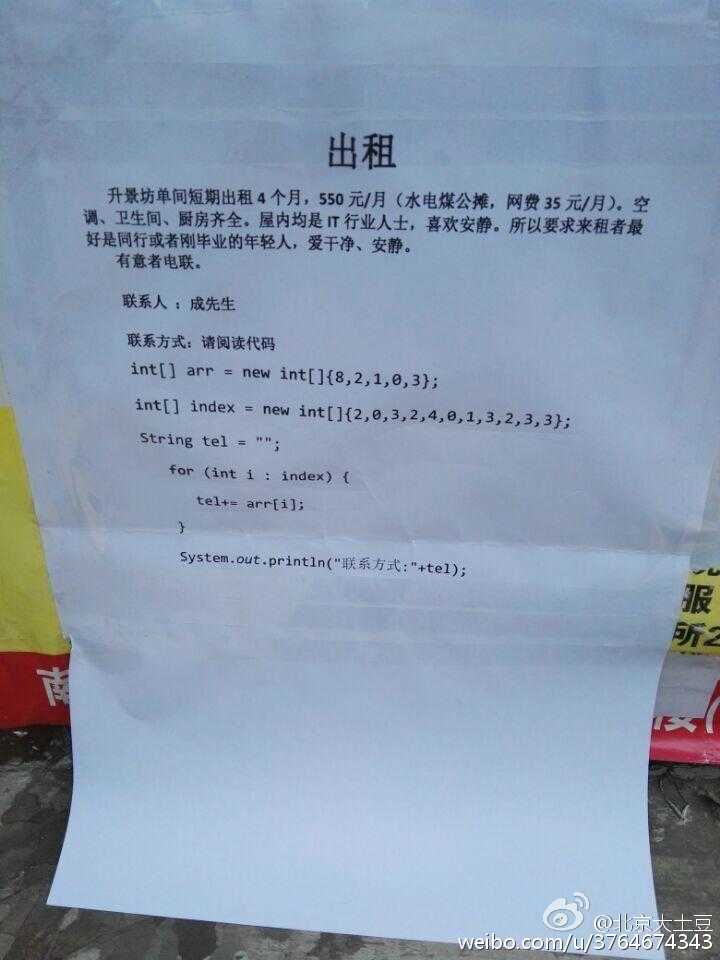设为首页 加入收藏
TOP
L1-027 出租
2019-02-12 18:08:00 】 浏览:101
 Tags：L1-027 出租

### 题目：18013820100

int[] arr = new int[]{8,3,2,1,0};
int[] index = new int[]{3,0,4,3,1,0,2,4,3,4,4};

### 上代码：

```#include <vector>
#include <iostream>
using namespace std;
int main()
{
string s;
cin>>s;
int count={0};
vector<int> arr;
vector<int> index;
for(int i=0;i<s.length();i++)
{
count[s[i]-'0']++;
}
for(int i=9;i>=0;i--)
{
if(count[i]!=0){
arr.push_back(i);
}
}
for(int i=0;i<s.length();i++)
{
for(int j=0;j<arr.size();j++)
{
if(s[i]-'0'==arr[j]){
index.push_back(j);
break;
}
}
}
cout<<"int[] arr = new int[]{"<<arr;
for(int i=1;i<arr.size();i++){
cout<<","<<arr[i];
}
cout<<"};"<<endl;
cout<<"int[] index = new int[]{"<<index;
for(int i=1;i<index.size();i++)
{
cout<<","<<index[i];
}
cout<<"};";
return 0;
}```

】【打印繁体】【投稿】【收藏】 【推荐】【举报】【评论】 【关闭】 【返回顶部